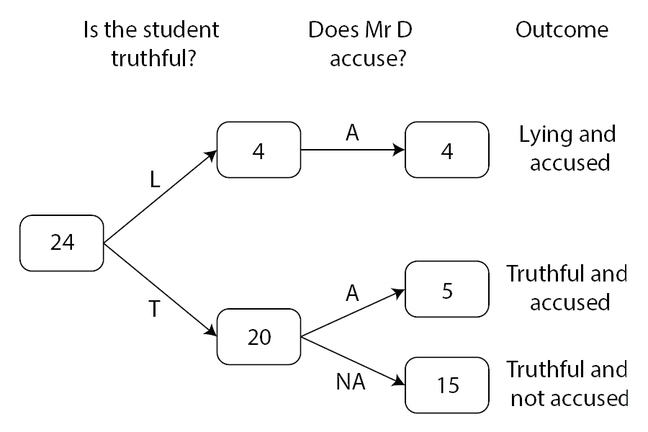## Want to keep learning?

This content is taken from the Cambridge University Press's online course, Teaching Probability. Join the course to learn more.
2.12

## Cambridge University PressWe can use expected frequency trees to solve problems

# Using frequency trees

We can use our expected frequency diagram to answer some key questions about the experiment.

We can clearly use the completed diagram above to answer some simple questions:

• What proportion of the students were lying?
• What proportion of the students were accused of lying?

We can see that 4 out of 24 students (or $$\frac{1}{6}$$) were lying, and that 9 out of 24 (or $$\frac{3}{8}$$) were accused of lying.

We can also consider the unfortunate wrongly accused students in two further ways:

• What proportion of the truthful students are accused?
• What proportion of the accused students are truthful?

These two questions are not the same. In each case, we first need to identify the reference class - that is, the set of students that we are finding a proportion of. In the first question, we are interested in the accused students who are a subset of the set of truthful students; in the second question, we are interested in the truthful students who are a subset of the set of accused students.

We can answer the first question as follows.

• There are 20 truthful students.
• Of those, five are accused.
• So the proportion of truthful students that are accused is 5/20 or $$\frac{1}{4}$$

The second question can be answered as follows:

• Nine students are accused altogether.
• Of those, 5 are truthful.
• So the proportion of accused students that are truthful is $$\frac{5}{9}$$

When we teach this activity, we introduce the following variation:

Mr L I Detector realises that his judgements are not always correct! How does it change the outcomes for the students if he does not automatically accuse people he suspects of lying?

In this version, all students are treated equally, whether Mr D suspects them of lying or not. In every case, the same spinner (three parts green, one part white) is used to decide what he does - he accuses students if, and only if, the spinner lands on white.

Using a new version of the template, can you complete the expected frequency diagram for this new situation? And can you then answer the same two questions for this variation?

• What proportion of the truthful students are accused?
• What proportion of the accused students are truthful?